## Monday, October 7, 2013

### A Collection of Interview Questions on Arrays

One dimensional array is probably the most important data types in programming languages. First of all, computer memory supports random access and can be treated as a big array. Second, other data structures can be implemented/simulated using arrays, e.g. strings, stacks, queues, binary heaps, linked lists, binary search trees, graphs can all be implemented using arrays. Because of this fundamentality, questions on arrays are commonly asked during a technical interview. The hardness level of such questions may vary from implementing a strcpy function to applying algorithmic tricks. In this post, I will try to cover a diverse set of these array questions, and I will keep this post updated when encountering new problems.

Coding interview questions usually test two aspects:

1. The ability to write correct and stylish programs. The interviewee should write syntactically correct C-family language code, and prove the correctness of the program by checking boundary conditions, loop invariants, etc. If the problem is relatively easy, not only the correctness but also the cleanness and readability of the code count.

2. The ability to think quickly and solve hard algorithmic problems. Google/Facebook/MSFT tend to ask tricky questions in their interviews. Most experienced programmers don’t think that using ACM/ICPC and algorithmic problems really test a programmer’s ability to code. However, this is a good way to see if the interviewee is clever or not (and clever people learn and adapt fast). More importantly, the coding of these problems will show how can you compact several coding tricks in, say, 20 lines of code.

Actually many problems here test both abilities, and in many cases writing correct programs with a good style weighted more than knowing the algorithmic trick to a specific problem.

Several problems in my collection are taken from a problem series by Chen Liren, link. If you read Chinese, I highly recommend this series, which contains many good questions and experience reports from real interviews.

The problem descriptions and some keywords use bold fonts.

Maximum subarray problem. This problem is so famous that it has its own Wikipedia page. It was popularized by Jon Bentley in his CACM column Programming Pearls and later collected in the book with the same name.

This problem can be solved in O(n) with O(1) space using dynamic programming. The DP solution itself is not very interesting. What interests me is two others solutions, one using a technique called cumulative sum, and the other using a more general technique -- divide and conquer.

Cumulative sum is a very useful pre-computing technique to allow O(1) range queries. It costs O(n^2) for the pre-computing thought.

Divide and conquer applies on a lot of sequence problems, including many sorting and searching algorithms. The trick is to design an O(n) merging step. In this problem O(n) merging is simple. In other problems, e.g. closest point pair, O(n) merging is non-trivial.

An extension of this problem is to find the maximum submatrix. An O(n^3) algorithm is expected.

Another variant of this problem: find two disjoints subarrays A and B such that |sum(A)-sum(B)| is maximized. Suppose A is on the left and B is on the right, for position i, we need the maximum/minimum subarray in range [1,i], and [i+1,n].

Given an array that is a rotation of a sorted array (elements are unique). The rotation of an array is to split the original array into two parts and concatenate the left part to the tail of the right part. E.g., {3,4,5,1,2} is a rotation of {1,2,3,4,5}. Now given this rotated array, find the smallest element.

Obviously we can do a linear scan and find the first number that is smaller than its left number. But linear scan costs O(n). Because the original array is sorted, it is natural to know binary search might get the running time to O(log n). In designing any binary search algorithm, it is important to prove that its correctness using loop invariant. [Bentley’s pearls and CLRS both cover loop invariant.] The algorithm sketch:

`l=1, r=nwhile a[l]>a[r] do  if (l+1==r) then    return r  m=(l+r)/2  if a[m]>a[l] then    l=m  else    r=mreturn a[l]`

Another binary search problem: given a sorted array, if A[i] == i, i is called magic index. Find the magic index.

Another binary search problem: given an array A=BC where B is a sorted array with non-increasing order, and C is a sorted array with non-decreasing order. Find B and C.

Not binary search, but incremental search: given an array A in which any two consecutive numbers diff by 1. Find the index of a target number T. E.g. A= {4,5,6,5,6,7,8,9,10,9}, and T=9.

Given an array of integers, write an algorithm that shuffles the array so that all odd numbers and ahead of even numbers.

This problem tests the understanding of quicksort algorithm. In quick sort’s partition procedure, once we fix a pivot, there is an O(n) algorithm that puts all elements less than or equal to the pivot to the left, and all elements that are greater to the right. In this problem, we shall redefine the comparison function that any odd number is “smaller” than any even number.

If C/C++ used, then write a general partition procedure which takes a comparison function pointer(C)/comparison operator(C++) as input would be nice.

A variant of this problem: 3-way partition. Given an array and i, design an in-place algorithm that puts all number < A[i] to the left, and all numbers = A[i] in the middle, and the rest in the right.

Another variant of partition algorithm: sort an array of 1/2/3 (don’t count # of 1,2,3), use a new partition algorithm instead.

Inversions. Given an array, calculates the numbers of pairs (i,j) such that A[i]>A[j].

This problem has several solutions. One is to modify the merge procedure in merge sort, to count the inversions in each merge procedure. Other solutions require using advanced data structures, and we don’t cover them here.

`long long res; void merge(int l, int m, int r){     int n1=m-l+1, n2=r-m;    for (int i=0; i<n1; i++) L[i]=a[l+i];     L[n1] = INF;     for (int i=0; i<n2; i++)  R[i]=a[m+i+1];     R[n2] = INF;     int j=0,k=0;     for (int i=l; i<=r; i++)         if (L[j]<=R[k]) a[i]=L[j++];         else { a[i]=R[k++]; res+=n1-j; } } void merge_sort(int l, int r){     if (l<r) {         int m=(l+r)/2;         merge_sort(l,m);         merge_sort(m+1,r);         merge(l,m,r);     } }`

Majority. Given an array of n integers, find the number that occurs more n/2 times, or report that such a number does not exist. E.g., in array {1,2,2,2,3}, 2 is such a number.

Solution 1: If such a number exists, then the n/2-th number in the sorted array must equal to it. Then we can first use a linear algorithm to find the n/2-th number in an unsorted array. In the literature, this k-th number problem is called selection problem, which has a complicated linear algorithm by Blum et. al. (four of the five authors of this paper were awarded Turing award!), and a O(n)-average time algorithm using quicksort variant.

Solution 2: a property of this problem: removing any two numbers that are different -> the frequent number remains the same. A walk through of the array using this property can get a candidate of the frequent number. Another linear scan checks whether the candidate occurs more than n/2 times.

A variant of this problem is to find a number that occurs more than n/3 times. The above two solutions still apply.

Given an array of integers, concatenate all numbers in some order so that the combined string is lexicological smallest. E.g., {3,32,321}, solution is 321323.

Define a new comparison operator of two numbers and sort the array. The new comparator is

`compare x y   if str(xy) > str(yx) then    1  else if str(xy) < str(yx) then    -1  else     0`

The tricky part is to prove that this does yield the smallest string. To prove this order is correct, we need to prove three properties: reflexive, symmetric and transitive.

Given a sorted array and an integer x, output the number of times this integer occurs in the array.

Linear scan costs O(n). A straightforward binary search can spot the first occurrence of this integer, and we continue to count until the next number is not equal to it. However, this integer can occur O(n) times. A workaround is to do binary search again at the first occurrence. Suppose the first occurrence is at position i, and there are m numbers to the right. First, we check position i + m/2, if equal, we check i + m/2 + m/4, if not equal, we check i + m/4, and so on. However, this is non-trivial to get correct because there are a few boundary conditions.

The best solution to this problem is to use two binary search functions in C++ STL: lower_bound and upper_bound. lower_bound finds the smallest position i such that A[i]>=x, upper_bound finds the smallest position i such that A[i]>x. The solution to this problem is simply upper_bound(x)-lower_bound(x). The two functions already take care of boundary conditions and cases where x does not occur in the array.

Given an array of integers, all integers occur exactly twice except for one. Find the only integer that occurs once.

Use bit trick. x xor x = 0. (if a bit occurs twice, set it to 0)

Variant: Given an array, except for one X, all other numbers occur exactly 3 times. Find X.

Now bit trick does not work. However, the idea is the same. If a bit occurs 3 times, set it to 0.

Integers and sum. Subproblem 1: given a sorted array, find two numbers that sum to m. Subproblem 2: the array is not sorted. Subproblem 3: find four numbers that sum to m.

For subproblem 1, there is a trick called ruler reading. Keep two pointers to the left and right of the array, and move the pointers until the sum if m. O(n)

For subproblem 2, hasing or sorting.

For subproblem 3, there is trick called two-head search. First, we get the sums of all pairs (O(n^2)), sort these sums or put them in a hash table. for a+b+c+d=m, we now only need to enumerate a and b, and check whether c+d exists using binary search or hashing.

Another problem using ruler reading technique: given an array of positive integers, and S. Find a subarray with minimal length and the sum of the subarray is >= S.

Arrange 0 to n-1 in a circle, starting from 0, delete every m-th number. Output the delete numbers in sequence. E.g, {0,1,2,3}, m=2, the sequence is 1,3,2,0.

Solution: O(n), in every step, use module operation to find the exact number.

Given an array of integers, find a maximum subset that constitutes a sequence of consecutive integers. E.g., {15, 7, 12, 6, 14, 13, 9, 11}, the answer is {11,12,…,15}.

Obvious solution is to sort the numbers in O(n log n) and then do linear scan. The solution requires a running time better than O(n log n). Hint: make the consecutive numbers into same set, and use disjoint set algorithms to have O(n log log n) running time.

Given k sorted arrays, find a range [a,b] with minimal length such that each array has at least one element in [a,b].

Example,

1: 4, 10, 15, 24, 26

2: 0, 9, 12, 20

3: 5, 18, 22, 30

Minimal range is [20,24].

If we have all the numbers in k arrays sorted, and mark the numbers with labels 1 to k, then our minimal range should contain all k labels. This range checking can actually be done while we do merge sort of the k arrays. Hint: set a pointer for each array.

Given an array of 0/1, find the longest subarray such that the number of 0s and the number of 1s are the same.

Using the cumulative sum technique, the problem can be solved in O(n^2). Hint: fill 0 with -1, and use cumulative sum technique again.

Largest Rectangle in a Histogram. Given an array of heights, calculate the rectangle with largest area.

Solution: pre-compute two arrays L and R, L[i] indicates the most left position where h[L[i]]>=h[i].The following C++ code snippet calculates L (R would be similar):

`int t = 0; for (int i=0; i<n; i++) { while (t>0 && h[st[t-1]] >= h[i]) t--;   L[i] = t==0?0:(st[t-1]+1);   st[t++]=i; }`

Many other solutions to this problem: http://www.informatik.uni-ulm.de/acm/Locals/2003/html/judge.html

Variant: Given an matrix with 0/1, find the max submatrix with only 1s.

A much easier problem: given an unsorted array A, find in linear time, i and j (j>i), such that A[j] – A[i] is maximized.

In-place shuffle array: 1, 2, 3, …, 2n => 1, n+1, 2, n+2, etc. solution: Peiyush Jain, A Simple In-Place Algorithm for In-Shu?e. http://arxiv.org/pdf/0805.1598.pdf

Coins change problem. Given an array A and X, find how many subsets of A sum to X. Solution: DP.

Given two arrays S and T. Each is a permutation of 0 to n-1. By swapping 0 and another number repeatedly, change S to T. If minimal swaps are required, how to do it?

Solution: get a position correct in every step. If minimal swaps are required, use BFS/short path algorithm.

Given an unsorted array, find the longest arithmetic progression. Case 1: keep order of the original array. Case 2: no need to keep order.

Solution:

For case 1: use this hash data structure to store the n(n-1)/2 pairs of difference:

map<int, set<pair<int, int> >

difference -> sorted set of (i,j) pairs [difference = a[j]-a[i] ]

For case 2: since the order does not matter, we first sort the array in O(nlogn), and then the longest arithmetic progression is a sub sequence of the sorted array. This can be solved by DP:

dp[i][j] = dp[j][k] + 1, if i<j<k, a[i]-a[j]=a[j]-a[k]

dp[i][n-1] = 2, for all i < n-1

Given an array of n unique numbers from 1 to n, delete 1/2/k numbers, how to identify the deleted number/numbers?

For k=1, calculate sum.

For k=2, calculate sum and sum of squares.

For k=3, calculate sum, sum of squares, and sum of cubes.

Time: O(nk)

Another solutions: count the 0/1 bit at each position. Time: O(n log n)

An array of length n+1 is populated with integers in the range [1, n]. Find a duplicate integer (just one, not all) in linear time with O(1) space. The array is read-only and may not be modified. Variation: what if the array may be written into but must be left unmodified by the algorithm?

Solution: http://gurmeet.net/puzzles/duplicate-integer/ and

The best solution requires solving several nontrivial subproblems, which are actually linked list problems!

Given an unsorted array A of n distinct natural numbers, find the first natural number that does not appear in the array.

I first saw this problem when reading R. Bird’s pearls of functional algorithm design, in which this problem is the first pearl. There are at least two good solutions (favor different tradeoffs) to this problem:

1. From 0 to n, there must be an integer that does not appear in A. We use an extra bool array with size n+1 and scan the array to mark occurred numbers. Then we can scan the bool array to find the first non-mark number as the solution. This solution is very easy to implement and costs O(n) time and O(n) space. Can we reduce the space cost?

2. Divide and conquer. Let’s check this property: from low to low+n, there must be a number that does not occur in A where low is the smallest (possible) number in A. We just need to guarantee that A does not have numbers smaller than low. Besides low, if we also know the upper bound value of A (we define upper bound as the number that is bigger than all numbers in A). So there are at most high-low distinct numbers in A. If and only if high-low==n, the numbers in [low, high) all occur in A once. If high-low>n, then there is a number in [low, high) that does not occur in A.

With this property, we can design a divide and conquer algorithm that in each step discard half of the numbers, the following is an implementation from Bird’s book:

`minfree xs          = minfrom 0 (length xs, xs)minfrom a (n, xs)   | n == 0    = a                    | m == b-a  = minfrom b (n-m, vs) -- discard the numbers < b                    | otherwise = minfrom a (m, us)   -- discard the numbers >= b                      where (us, vs) = partition (< b) xs  -- partition in quicksort                            b        = a + 1 + n div 2                            m        = length us`

This method uses O(n) time and O(log n) space. But the space cost can be reduced to O(1) if we write a iterative version of the above procedure. And different from linear selection algorithm which is only O(n) on average case, the algorithm here is strictly O(n).

Two problems on permutation arrays:

Find the next permutation. This function is implemented in C++ STL. Its Wikipedia page has a solution. The following is an OCaml implementation:

`let next_permutation a =     let swap i j =          let tmp = a.(i) in                  a.(i) <- a.(j);         a.(j) <- tmp;     in     let n = Array.length a in     let k = ref 0 in    let i = ref (-1) in     begin          (* find largest i such that a(i) < a(i+1) *)         while !k<n-1 do             if a.(!k) < a.(!k + 1) then                 i := !k;             k := !k + 1         done;         if !i = -1 then None (* if such i does not exist, no next permutation *)         else begin             (* find largest j such that a(j) > a(i) *)             let k = ref (!i + 1) in             let j = ref !k in            while !k<n do                if a.(!k)>a.(!i) then                     j := !k;                 k := !k + 1             done;             (* swap i j *)             swap !i !j;             (* reverse all after index i *)             let k1 = ref (!i + 1) in             let k2 = ref (n - 1) in             while !k1 < !k2 do                swap !k1 !k2;                 k1 := !k1 + 1;                 k2 := !k2 - 1;             done;             Some a;         end     end`

Find the inverse of a permutation. E.g. A = [4,2,1,3] is a permutation, its inverse is B = [3,2,4,1], i.e. B[A[i]] = i (index starts from 1).

Solution: inverse several circle linked lists.

# Problems with no good solutions yet:

Given an array A with length n, filled with 1 to n. Some numbers occur multiple times, some don’t occur. Identify these two groups of numbers in O(n) time and O(1) space.

Given an array, some numbers occur once, some twice, and only one X three time. Find X. (No solution yet)

## Sunday, September 22, 2013

### Kindle Wordbook -- Making a Personalized Dictionary from Books You’ve Read

Kindle Paperwhite is really a good e-reader. Since I had it I have read and re-read several books with great joy and ease. As I am not a native English speaker, I rely on the dictionary function in Paperwhite. Of course, this dictionary looking function is implemented similarly in other software running on Android and iOS. However I found Paperwhite offers the best touch experience (In the appendix of the article, I compare the dictionary looking function in Paperwhite with iBooks and Duokan reader). Paperwhite is also more suitable for reading plain words than a tablet/phone, in terms of light, size, and battery life.

Kindle stores all the highlighted texts, bookmarks, and notes in a text file: My Clippings.txt. When I look one word in the dictionary, I will immediately highlight it. This highlighting action does not cost any extra touch because one touch is anyway needed to close the dictionary window.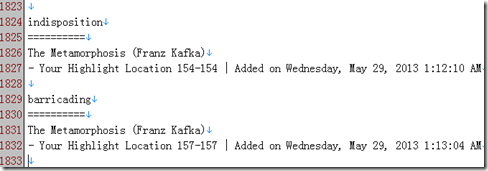Figure 1. My Clippings.txt opened in a text editor.

One problem with highlighted words is that they are just words -- they don’t carry the meanings in the dictionary, nor do they contain the context of the highlighted words. For many words that I have looked up before, when I later encounter them again, I still could not remember their meanings.

So my idea is to parse My Clippings.txt and find the highlighted words out and then

1) find the Chinese meanings of them using an online dictionary, and

2) find all occurrences in my past readings.

For the first task, I can use the API of an online dictionary. After some searching, I find www.wordreference.com provides a simple API. An alternative is dict.baidu.com, which can be accessed via HTTP requests.

If I just aim to get the Chinese meanings of the unknown words, then I only need to do the first task. However by merely looking at the Chinese translations of the words, I may easily forget some of them; and even I remember the vague meanings of these words, I still don’t know when and how to use these words. The vocabulary that we apply in writing and speaking is much less than that we can recognize while reading. By reviewing these words in their context, I can remember them better, and more importantly, by learning their usages in different texts by different authors, I can summarize when and how they are used. For example, I get to know the word `remorse’ while reading Mary Shelley’s Frankenstein and this word is then linked with various episodes and images in the novel. The word not only has a meaning, but has a more concrete feeling. Acquiring such a concrete feeling, I think, is a perquisite towards using the word confidently and correctly.

So I wrote a little Python program to do the two tasks automatically for me. The program can be downloaded at:

https://github.com/yinz/pwdict

And have a look at my recent words in browser: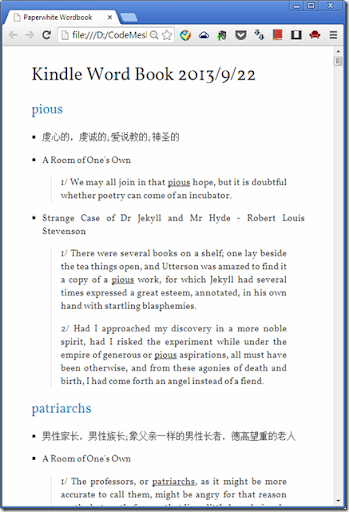Figure 2. View word book in a browser.

Python is very good at trying out ideas and making things done quickly because of its vast libraries and dynamic nature. In this project, I need HTTP requests, parsing json text, reading epub files, and parsing English sentences. There are libraries for all these tasks in Python. The HTTP and json libraries are built-in. And I modified epub2txt project to extract the pure text content from epub formats, which are typical book formats used across various mobile reading software. Parsing an article into individual sentences seems easy, but can be tricky in many special cases. So I used the NLTK library for this.

# Appendix. Why dictionary looking in Paperwhite is better

Paperwhite: one touch to open dictionary, and one touch to back (marking the word does not cost extra touches)

Duokan and iBooks: two touches to open dictionary, and one touch to go back. Marking the word cost extra two touches!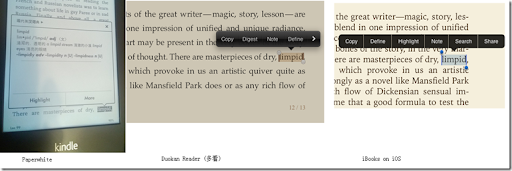## Wednesday, July 17, 2013

### Subtle Variable Scoping in R

A languages manual usually defines how a language behaves, but does not warn you in cases where you assume a feature should be supported but isn’t. As an example, I will talk about the subtle variable scoping in R language.

# {} code blocks

A lot of programmers coming from C/C++/Java will assume that code blocks inside {} also introduce a new scope. However, in dynamic languages like R/Python/JavaScript/Matlab, code blocks do not introduce new scopes; only function does. This difference may cause some subtle bugs.

For example, the following R function returns a list of quadratic function objects:

`make_funcs <- function(a, b, c_){  n <- length(a)  fs <- list()  for (i in 1:n) {    fs[[i]] <- function(x) {      cat(sprintf('eval %.1f*x^2+%.1f*x+%.1f where x = %.1f\n', a[i], b[i], c_[i], x))      a[i]*x*x + b[i]*x + c_[i]    }  }  cat(sprintf('variable i is still in the scope and has value %d\n', i))  fs}`

The input to this functions is three vectors of numbers which represent the three coefficients in quadratic forms. And let’s make three objects using the following coefficients:

`a <- c(1,2,3)b <- c(4,5,6)c_ <- c(-1,-1,-1)`

`fs <- make_funcs(a, b, c_)fs[](1) fs[](1) fs[](1) `

We are supposed to get three different function values. However, all the three functions are the same after checking the output:

`> fs[](1)eval 3.0*x^2+6.0*x+-1.0 where x = 1.0 8> fs[](1)eval 3.0*x^2+6.0*x+-1.0 where x = 1.0 8> fs[](1)eval 3.0*x^2+6.0*x+-1.0 where x = 1.0 8`

It seems that the three fs[i] use the same variable i when they are evaluated. That is, when the three functions are created, the R interpreter just remembers i as a variable in its parent function. Then the result can be explained: after the loop is finished, the variable i has value of 3, and it is still inside the scope of make_func.

Let’s see how will we write make_func in F#:

`// a direct translation let make_funcs (a: int array, b:int array, c: int array) =    let n = Array.length a    let fs = new ResizeArray<(int -> int)>()    for i=0 to n-1 do        fs.Add(fun x -> a.[i]*x*x + b.[i]*x + c.[i])    fs.ToArray()// a more functional translation let make_funcs2 (a: int array, b:int array, c: int array) =    Array.zip3 a b c    |> Array.map (fun (a0, b0, c0) ->         (fun x -> a0*x*x + b0*x + c0))`

The following code would make three different functions as we expect:

`let a = [| 1; 2; 3 |]let b = [| 4; 5; 6 |]let c = [| -1; -1; -1 |]let fs = make_funcs (a, b, c)fs.(1) // 4fs.(1) // 6fs.(1) // 8`

Why F# code works as expected? When the three functions are created, they also know that variable i shall be found in the parent scope; however the three is have three independent scopes!

As the behavior in R’s version is definitely not what we want. How to make three different functions? Answer is make three different is in side a new function:

`make_funcs2 <- function(a, b, c_){  n <- length(a)  fs <- list()  for (i in 1:n) {    fs[[i]] <-      (function() {        j <- i        function(x) {          cat(sprintf('eval %.1f*x^2+%.1f*x+%.1f where x = %.1f\n', a[j], b[j], c_[j], x))          a[j]*x*x + b[j]*x + c_[j]        }       }) ()  }  fs}`

Each time in the for loop, I create a new function and defines a variable j inside it, and the new function return the function(x). Notice that this new function is executed for n times in the for loop, therefore creates n different js.

This trick is ubiquitously used in JavaScript. For example instead of writing,

`{  var a = 1;  //code blocks}`

we define a function and execute it immediately to make local variables:

`(function() {  var a_is_hidden_from_outside = 1;  // in other words, no new variable in the global space is introduced. }) ()`

# The assignment operators <- and <--

It seems that the block syntax {} can be translated as (function() {}) () in R/JavaScript. But in R, things can be more subtle. See the third version of make_funcs:

`make_funcs3 <- function(a, b, c_){  n <- length(a)  fs <- list()  for (i in 1:n)       (function() {        j <- i        fs[[i]] <-          function(x) {            cat(sprintf('eval %.1f*x^2+%.1f*x+%.1f where x = %.1f\n', a[j], b[j], c_[j], x))            a[j]*x*x + b[j]*x + c_[j]          }        print(length(fs))      }) ()    fs}a <- c(1,2,3)b <- c(4,5,6)c_ <- c(-1,-1,-1)fs <- make_funcs3(a, b, c_)fs[](1)`

We translate {} after for loop as (function () {}) (), and now the assignment of fs[i] is inside the function wrapper. However, the code would not run correctly:

`> fs <- make_funcs(a, b, c_)ls length = 1ls length = 2ls length = 3> > fs[](1)Error in fs[] : subscript out of bounds> length(fs) 0`

Obviously the variable fs is growing when the for loop is executed, however the variable fs inside the for loop is a different one from the one outside the for loop. And we find that the variable fs outside the for loop is only initialized but has not been added any new elements.

The assignment operator <- creates a new variables inside a function! It won’t search if the same variable name is in its parent environments! To do that which is what we suppose R to do, we have to use the <<- operator:

`make_funcs3 <- function(a, b, c_){  n <- length(a)  fs <- list()  for (i in 1:n)       (function() {        j <- i        fs[[i]] <<-          function(x) {            cat(sprintf('eval %.1f*x^2+%.1f*x+%.1f where x = %.1f\n', a[j], b[j], c_[j], x))            a[j]*x*x + b[j]*x + c_[j]          }        cat(sprintf('ls length = %d\n', length(fs)))      }) ()  fs}`

And now this function should run as we expected.

# Summary

In using R for interactive data analysis and plotting, most of time we won’t deal with these subtle language features. We just copy/paste some code snippet from R help and online and modify it to suit our own data analysis. However when we are into R programming, these issues do occur and will bite us when we assume our experience in C++/Java would also work in R.

## Tuesday, July 9, 2013

### Unicode Tips in Python 2 and R

Most of time, I don’t need to deal with different encodings at all. When possible, I use ASCII characters. And when there is a little processing in Chinese characters or other Unicode characters, I use .Net languages or JVM languages, in which every string is Unicode and of course when the characters are displayed they are displayed as characters (not as the unreadable escaped strings or Unicode IDs).

However recently I am working on projects on Chinese Weibo data, and I encountered some Unicode problems when using Python and R. (I use Python for data crawling and processing and R for modeling and visualization.)

This post is a note I start today and I will update it when I encounter new Unicode problems…

# Python 2.7

Lesson 1: Normal string and Unicode string are two types. Do not mix use them.

When writing Unicode strings literals, we put a prefix u before the string:

`>>> type(u'')<type 'unicode'>>>> type('')<type 'str'>`

Notice that the types of the two strings are different, one is 'unicode’, and the other is ‘str’. Python is a dynamic language and sometimes will do smart things between the two types. But we should use Python as if it is a strict static type language and never cross use the two types, and when needed, do conversions explicitly. See the following example:
`>>> u'abcd'.find('b')1>>> u'你好'.find('好')Traceback (most recent call last):  File "", line 1, in     u'你好'.find('好')UnicodeDecodeError: 'ascii' codec can't decode byte 0xba in position 0: ordinal not in range(128)`

The first find operation works fine because it does not involve any non-ascii characters. The second won’t work because the two types don’t work with each other.

The error in the example seems simple to avoid. However when you load a set of text files from different sources, you may mix Unicode strings and normal strings.

Lesson 2: When passing a Unicode string to a function not written by you, check carefully whether that function supports Unicode.

It seems that if we have all the strings as Unicode strings in Python 2.7, we won’t have any problems. We can add u prefix to all non-ascii string in the file, and we also use codecs package to read Unicode files:

`    with codecs.open(unicode_text_file, 'r', encoding='utf-8') as f:        read the file and all strings you get are of unicode type`

Everything seems fine until we call function/packages that are written only for normal strings and we wished they would work for Unicode strings. For example, the csv package in Python 2.7 official release does not work with Unicode files. You cannot do things like:

`    with codecs.open(file_profile, 'r', encoding='utf-8') as f:        reader = csv.reader(f)        head = reader.next()`

The third line will throw UnicodeDecodeError just as above. So in Python 2.7 this is the pain point – you cannot expect all the common libraries that work nicely with normal strings to work nicely with Unicode strings. And a script that worked before for ascii files can suddenly fail on a Unicode file.

For library writers, it is pain too. Sometimes they need to write a special version for Unicode. I once worked with a human name parser in Python. European names can have accents on letters, but that library only accepts ascii strings. To use that name parser, I have to

1. Convert the name string into a normal Python string by removing all accents

2. Parse it using the library

3. Convert the result to Unicode string using .decode method

# R

Lesson 1: In R, there is only one type of string, that is character. But this type can store whatever can be repressed as byte arrays. Think of R’s character type as C’s char[] and nothing more.

In Python, we have two types for two kinds of strings. In R, we have only one type for all kinds of strings.

My first problem is displaying Unicode characters in R. R’s default GUI and command line console cannot even display Chinese on a non-Chinese Windows. (On a Chinese Windows, you can only get a Chinese version R. Chinese characters are fine. However all info/error messages are also in Chinese, which are translated from English and which are just wired Chinese.)

When R is installed, it checks the System encoding. On Linux and Mac OS, the system encoding is usually UTF-8, and R uses that. However Windows is different, the following is my R session info:

`> sessionInfo()R version 2.15.1 (2012-06-22)Platform: x86_64-pc-mingw32/x64 (64-bit)locale: LC_COLLATE=English_United States.1252  LC_CTYPE=English_United States.1252    LC_MONETARY=English_United States.1252 LC_NUMERIC=C                           LC_TIME=English_United States.1252    `

R’s default GUI then uses the encoding to display all strings. Of course, it fails on most Unicode characters.

Luckily the editor and the console in RStudio can display Chinese characters. And RStudio must have done some smart things:

`> as.character(china_mapdata\$NAME) "黑龙江省"> "黑龙江省" "黑龙江省"> as.character(china_mapdata\$NAME) == "黑龙江省" FALSE`

The string in the data frame displays the same as the literal (the literal is represented as Unicode in RStudio console), yet they are not equal in many ways. This is because two strings display the same can be different in their internal representations:

`> nchar("黑龙江省") 4> nchar(as.character(china_mapdata\$NAME)) 8`

Their types are both character. However the representation are different: the literal is represented as 4 Unicode characters (4 chars * 3 bytes/char = 12 bytes) in the memory, and the string read from the data file is represented as 8 bytes (4 chars * 2 bytes/char = 8 bytes) in the memory:

`> charToRaw("黑龙江省")  e9 bb 91 e9 be 99 e6 b1 9f e7 9c 81> charToRaw(as.character(china_mapdata\$NAME)) ba da c1 fa bd ad ca a1`

I find that the Chinese characters in the file I load the data frame uses GB2312 encoding. And because it is a binary file, I don’t have a simple way to change its encoding. But here is a method that I find:

`# First write the data frame to diskwrite.csv(china_mapdata, file = 'china_map.csv')# In EmEditor, open it as GB2312, and SaveAs UTF-8# Load the utf-8 filechina_mapdata <- read.csv('china_map.utf8.csv', encoding='UTF-8')# Testas.character(china_mapdata\$NAME) == "黑龙江省" # should be TRUE now`

After converting all Chinese characters in Unicode, I can now follow the map example here (the author of the post, I believe, uses a Chinese Windows, therefore does not have my problem; All systems except Chinese Windows will encounter the coding problem though) :

`ggplot(zhejiang, aes(x = long, y = lat, group = group,fill=NAME))+  geom_polygon(fill="beige" )+  geom_path(colour = "grey40")+  ggtitle("中华人民共和国浙江省")+  geom_point(x=120.12,y=30.16,fill=FALSE)+  annotate("text",x=120.3,y=30,label="杭州市")`

But when exporting the file as PDF, the Chinese characters cannot display correctly: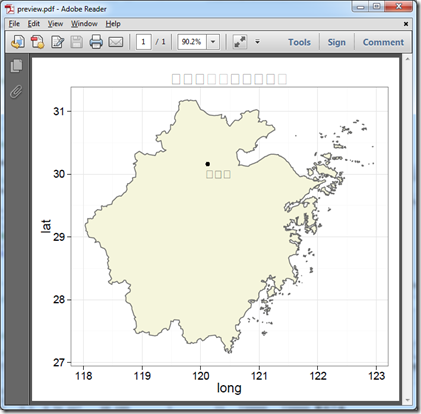After searching online, I find the solution in this SO question, and specifying the font explicitly solves the problem:

`cairo_pdf("example.pdf", family="FangSong")ggplot(zhejiang, aes(x = long, y = lat, group = group,fill=NAME))+  geom_polygon(fill="beige" )+  geom_path(colour = "grey40")+  ggtitle("中华人民共和国浙江省")+  geom_point(x=120.12,y=30.16,fill=FALSE)+  annotate("text",x=120.3,y=30,label="杭州市")dev.off()`

The correct PDF output: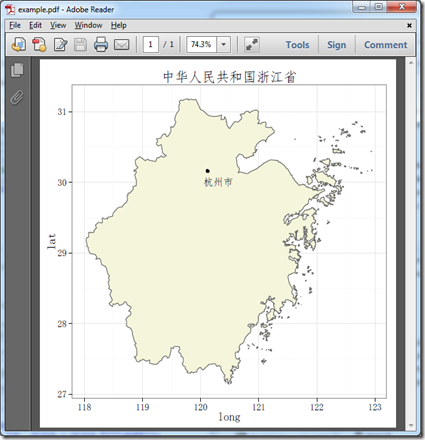We can also change the font type to other Chinese fonts, refer their names here.

Some R’s functions can recognize the Unicode string, e.g. Encoding. I think such recognition is based on the first few bits of the string. But it does not recognize GB2312 string (Encoding function outputs ‘unknown’ for GB2312 strings). Magically RStudio in Windows (English version, locale set to Simplified Chinese) can recognize both strings and display them correctly.

Lesson 2: When dealing with Unicode in R, use a Chinese Windows (for Chinese only), or use Linux/Mac OS (which is by default UTF-8), otherwise you cannot display Unicode well.

See how Unicode code may be displayed in R's console (English Windows):

`> head(zhejiang)    X.U.FEFF.  AREA PERIMETER BOU2_4M_ BOU2_4M_ID ADCODE93 ADCODE99                     NAME223       222 9.277    26.825      224         33   330000   330000 <u  +6D59><u  +6C5F><u  +7701>224       223 0.000     0.103      225       1626   330000   330000 <u  +6D59><u  +6C5F><u  +7701>225       224 0.000     0.052      226       1634   330000   330000 <u  +6D59><u  +6C5F><u  +7701>`

The <u+xxx> symbols indicate that R knows that they are Unicode characters, however R cannot display them correctly. And these symbols occur as symbols in your plots as well, which is just meaningless. If R cannot display these characters as characters, how can we know what are they and how to use these characters in a plot?

To solve this problem, my solution is to use a Linux with UTF-8 encoding. This is the session info in my Linux virtual machine:

`> sessionInfo()R version 2.14.1 (2011-12-22)Platform: i686-pc-linux-gnu (32-bit)locale:  LC_CTYPE=en_US.UTF-8       LC_NUMERIC=C               LC_TIME=en_US.UTF-8        LC_COLLATE=en_US.UTF-8      LC_MONETARY=en_US.UTF-8    LC_MESSAGES=en_US.UTF-8    LC_PAPER=C                 LC_NAME=C                   LC_ADDRESS=C               LC_TELEPHONE=C             LC_MEASUREMENT=en_US.UTF-8 LC_IDENTIFICATION=C       `

# Summary

Conceptually Unicode in Python and R are quite simple. In Python, Unicode and normal strings have different types. In R, all the strings are represented as byte arrays (the good old C char[]!); so from its type, you cannot decide whether a string is Unicode or not. I think Python’s two-type design is good because the runtime exception will force the programmer to split normal strings and Unicode strings clearly (though it is headache, this is why Python 3 treat all strings as Unicode strings) .

What adds to the Unicode complexity is functions that manipulate Unicode strings and especially the display of Unicode characters on screens. A Unicode character is anyway 2-4 bytes in the memory, nothing special. When using Python and R for data analysis, we usually follow the REPL (Read–eval–print loop), it is meaningless to display Unicode as escaped strings. One cannot even judge whether the character is a Chinese character by reading the escaped strings! What’s worse is that different terminals may display differently for the same character. For example, in a Unicode-enabled Python console:

`>>> print u'你'你>>> u'你'u'\u4f60'`

In a non-Unicode console:

`>>> u'你'u'\xc4\xe3'>>> print u'你'Äã>>> print u'\u4f60'你>>> u'你' == u'\u4f60'False(In this example, the problem is not that the terminal cannot display Unicode characters, it is that the input characters in the terminal are not Unicode!)`

The suggestion for the terminal and editor is

Use a Unicode terminal and a Unicode text editor when working with Python and R. For example, RStudio is, while Rgui.exe isn’t. PyDev plugin/PyScripter is, while the default IDLE isn’t.

And in Python, always put

`# -*- coding: utf-8 -*-`

at the beginning of the file, and save all source code files as Unicode.

## Monday, January 28, 2013

### A simple GUI tool to help find the right people

Today our professor asked us to find a list of researchers who are suitable as program committees for the big data conference:

2013 IEEE International Conference on Big Data (IEEE BigData 2013)

IEEE BigData is a brand new conference and does not have a “circle” yet. That means finding PCs is harder than other more established conferences. Our solution is to search the PCs in other major conferences such as WWW/KDD and find researchers who are doing big data.

After I count the number of PCs in SIGIR conference, I know that I need a tool to help me. SIGIR’11 has 223 researchers in its community! Think about repeating the following tasks for 223 times: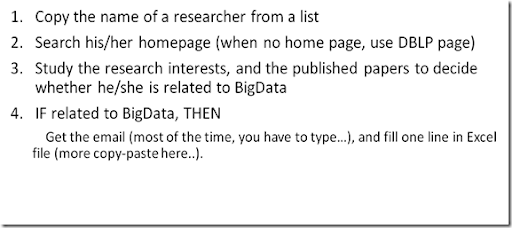I want to automate the following the tasks: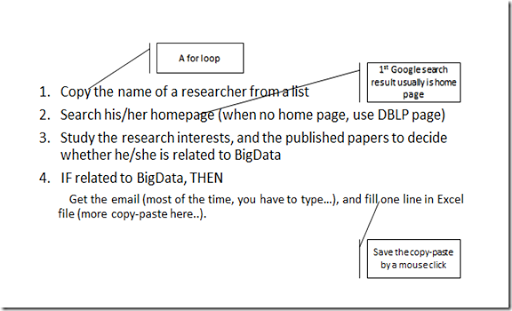There are two tasks that are hard:

1. Getting emails automatically. Some researchers simply don’t write emails on their webpages. To find the email, one have to open one of their research papers.

2. Decide if a researcher is related to big data. Actually we can formulate the problem into a binary classification task, which however requires some training data. So we go back to human labeling. Currently I define a set of keywords that indicate BigData:

let keywords =
[ "large"
"scale"
"big"
"data"
"mapreduce"
"distributed"
"system"
"cloud"
"computing"
"parallel"
]

and for each home page, I list the subset of keywords that occur in it. If no keyword is in his home page, then it is unlikely the researcher is doing something related to BigData. So the keyword filter is served as a helper for the human to decide.

I write an F# program and the code can be find on BitBucket. Here is a screen shot of running program: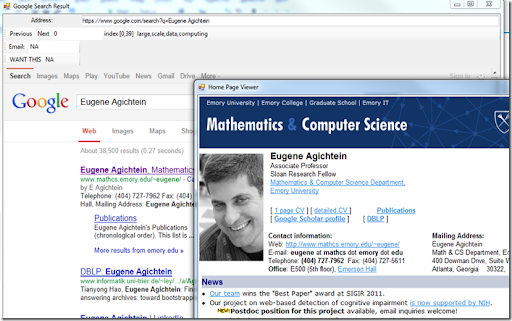When I click “WANT THIS”, the program inserts a record to the log file.

There two windows, one is for Google search and the other is for the page of the first search result. I keep two pages because occasionally the first result is not correct, I can then click other search results.

Some technical details:

1. WebBrowser in F# with only four lines!

let form = new Form(Visible=true, Text="Google Search Result")
let content = new WebBrowser()